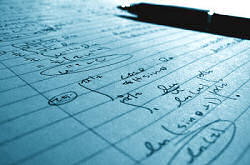Search IntMath
Close

# Methods of Integration

By M BourneImage source: Akash_k.

### Related Sections in "Interactive Mathematics"

Introduction to Integration

Applications of Integration

Fourier Series application

Differential Equations are a special type of integration

Laplace Transforms of Integrals

This chapter explores some of the techniques for finding more complicated integrals. (If you need to go back to basics, see the Introduction to Integration.)

By studying the techniques in this chapter, you will be able to solve a greater variety of applied calculus problems.

Some of the techniques may look a bit scary at first sight, but they are just the opposite of the basic differentiation formulas and transcendental differentiation formulas.

Also, the methods in this chapter are based on the General Power Formula for Integration which we met before.

Good luck!

You may be interested to read the Introduction to Calculus, which has a brief history of calculus.

## In this Chapter

We begin with a fundamental technique, the General Power Formula »

## Problem SolverThis tool combines the power of mathematical computation engine that excels at solving mathematical formulas with the power of GPT large language models to parse and generate natural language. This creates math problem solver thats more accurate than ChatGPT, more flexible than a calculator, and faster answers than a human tutor. Learn More.